# 1.3.2. Numerical operations on arrays¶

## 1.3.2.1. Elementwise operations¶

### 1.3.2.1.1. Basic operations¶

With scalars:

>>> a = np.array([1, 2, 3, 4])
>>> a + 1
array([2, 3, 4, 5])
>>> 2**a
array([ 2,  4,  8, 16])


All arithmetic operates elementwise:

>>> b = np.ones(4) + 1
>>> a - b
array([-1.,  0.,  1.,  2.])
>>> a * b
array([ 2.,  4.,  6.,  8.])

>>> j = np.arange(5)
>>> 2**(j + 1) - j
array([ 2,  3,  6, 13, 28])


These operations are of course much faster than if you did them in pure python:

>>> a = np.arange(10000)
>>> %timeit a + 1
10000 loops, best of 3: 24.3 us per loop
>>> l = range(10000)
>>> %timeit [i+1 for i in l]
1000 loops, best of 3: 861 us per loop


Warning

Array multiplication is not matrix multiplication:

>>> c = np.ones((3, 3))
>>> c * c                   # NOT matrix multiplication!
array([[ 1.,  1.,  1.],
[ 1.,  1.,  1.],
[ 1.,  1.,  1.]])


Note

Matrix multiplication:

>>> c.dot(c)
array([[ 3.,  3.,  3.],
[ 3.,  3.,  3.],
[ 3.,  3.,  3.]])


Exercise: Elementwise operations

• Try simple arithmetic elementwise operations: add even elements with odd elements
• Time them against their pure python counterparts using %timeit.
• Generate:
• [2**0, 2**1, 2**2, 2**3, 2**4]
• a_j = 2^(3*j) - j

### 1.3.2.1.2. Other operations¶

Comparisons:

>>> a = np.array([1, 2, 3, 4])
>>> b = np.array([4, 2, 2, 4])
>>> a == b
array([False,  True, False,  True], dtype=bool)
>>> a > b
array([False, False,  True, False], dtype=bool)


Tip

Array-wise comparisons:

>>> a = np.array([1, 2, 3, 4])
>>> b = np.array([4, 2, 2, 4])
>>> c = np.array([1, 2, 3, 4])
>>> np.array_equal(a, b)
False
>>> np.array_equal(a, c)
True


Logical operations:

>>> a = np.array([1, 1, 0, 0], dtype=bool)
>>> b = np.array([1, 0, 1, 0], dtype=bool)
>>> np.logical_or(a, b)
array([ True,  True,  True, False], dtype=bool)
>>> np.logical_and(a, b)
array([ True, False, False, False], dtype=bool)


Transcendental functions:

>>> a = np.arange(5)
>>> np.sin(a)
array([ 0.        ,  0.84147098,  0.90929743,  0.14112001, -0.7568025 ])
>>> np.log(a)
array([       -inf,  0.        ,  0.69314718,  1.09861229,  1.38629436])
>>> np.exp(a)
array([  1.        ,   2.71828183,   7.3890561 ,  20.08553692,  54.59815003])


Shape mismatches

>>> a = np.arange(4)
>>> a + np.array([1, 2])
Traceback (most recent call last):
File "<stdin>", line 1, in <module>
ValueError: operands could not be broadcast together with shapes (4) (2)
Traceback (most recent call last):
File "<stdin>", line 1, in <module>
ValueError: operands could not be broadcast together with shapes (4) (2)


Transposition:

>>> a = np.triu(np.ones((3, 3)), 1)   # see help(np.triu)
>>> a
array([[ 0.,  1.,  1.],
[ 0.,  0.,  1.],
[ 0.,  0.,  0.]])
>>> a.T
array([[ 0.,  0.,  0.],
[ 1.,  0.,  0.],
[ 1.,  1.,  0.]])


Warning

The transposition is a view

As a results, the following code is wrong and will not make a matrix symmetric:

>>> a += a.T


It will work for small arrays (because of buffering) but fail for large one, in unpredictable ways.

Note

Linear algebra

The sub-module numpy.linalg implements basic linear algebra, such as solving linear systems, singular value decomposition, etc. However, it is not guaranteed to be compiled using efficient routines, and thus we recommend the use of scipy.linalg, as detailed in section Linear algebra operations: scipy.linalg

Exercise other operations

• Look at the help for np.allclose. When might this be useful?
• Look at the help for np.triu and np.tril.

## 1.3.2.2. Basic reductions¶

### 1.3.2.2.1. Computing sums¶

>>> x = np.array([1, 2, 3, 4])
>>> np.sum(x)
10
>>> x.sum()
10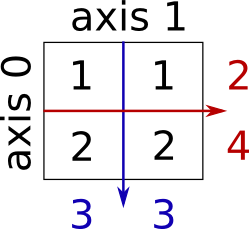Sum by rows and by columns:

>>> x = np.array([[1, 1], [2, 2]])
>>> x
array([[1, 1],
[2, 2]])
>>> x.sum(axis=0)   # columns (first dimension)
array([3, 3])
>>> x[:, 0].sum(), x[:, 1].sum()
(3, 3)
>>> x.sum(axis=1)   # rows (second dimension)
array([2, 4])
>>> x[0, :].sum(), x[1, :].sum()
(2, 4)


Tip

Same idea in higher dimensions:

>>> x = np.random.rand(2, 2, 2)
>>> x.sum(axis=2)[0, 1]
1.14764...
>>> x[0, 1, :].sum()
1.14764...


### 1.3.2.2.2. Other reductions¶

— works the same way (and take axis=)

Extrema:

>>> x = np.array([1, 3, 2])
>>> x.min()
1
>>> x.max()
3

>>> x.argmin()  # index of minimum
0
>>> x.argmax()  # index of maximum
1


Logical operations:

>>> np.all([True, True, False])
False
>>> np.any([True, True, False])
True


Note

Can be used for array comparisons:

>>> a = np.zeros((100, 100))
>>> np.any(a != 0)
False
>>> np.all(a == a)
True

>>> a = np.array([1, 2, 3, 2])
>>> b = np.array([2, 2, 3, 2])
>>> c = np.array([6, 4, 4, 5])
>>> ((a <= b) & (b <= c)).all()
True


Statistics:

>>> x = np.array([1, 2, 3, 1])
>>> y = np.array([[1, 2, 3], [5, 6, 1]])
>>> x.mean()
1.75
>>> np.median(x)
1.5
>>> np.median(y, axis=-1) # last axis
array([ 2.,  5.])

>>> x.std()          # full population standard dev.
0.82915619758884995


... and many more (best to learn as you go).

Exercise: Reductions

• Given there is a sum, what other function might you expect to see?
• What is the difference between sum and cumsum?

Worked Example: data statistics

Data in populations.txt describes the populations of hares and lynxes (and carrots) in northern Canada during 20 years.

You can view the data in an editor, or alternatively in IPython (both shell and notebook):

In : !cat data/populations.txt


First, load the data into a Numpy array:

>>> data = np.loadtxt('data/populations.txt')
>>> year, hares, lynxes, carrots = data.T  # trick: columns to variables


Then plot it:

>>> from matplotlib import pyplot as plt
>>> plt.axes([0.2, 0.1, 0.5, 0.8])
>>> plt.plot(year, hares, year, lynxes, year, carrots)
>>> plt.legend(('Hare', 'Lynx', 'Carrot'), loc=(1.05, 0.5))The mean populations over time:

>>> populations = data[:, 1:]
>>> populations.mean(axis=0)
array([ 34080.95238095,  20166.66666667,  42400.        ])


The sample standard deviations:

>>> populations.std(axis=0)
array([ 20897.90645809,  16254.59153691,   3322.50622558])


Which species has the highest population each year?:

>>> np.argmax(populations, axis=1)
array([2, 2, 0, 0, 1, 1, 2, 2, 2, 2, 2, 2, 0, 0, 0, 1, 2, 2, 2, 2, 2])


Worked Example: diffusion using a random walk algorithmTip

Let us consider a simple 1D random walk process: at each time step a walker jumps right or left with equal probability.

We are interested in finding the typical distance from the origin of a random walker after t left or right jumps? We are going to simulate many “walkers” to find this law, and we are going to do so using array computing tricks: we are going to create a 2D array with the “stories” (each walker has a story) in one direction, and the time in the other: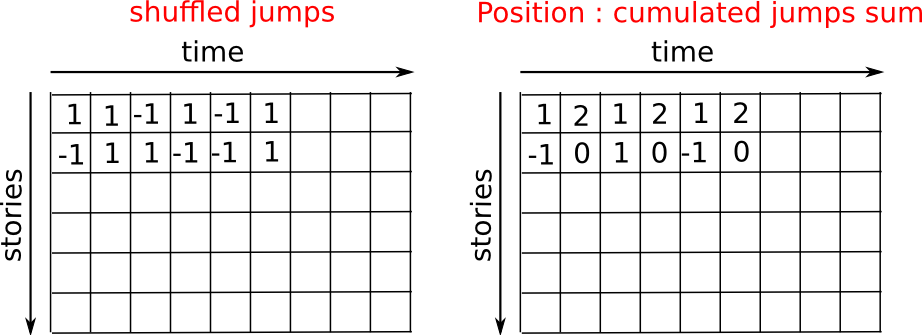>>> n_stories = 1000 # number of walkers
>>> t_max = 200      # time during which we follow the walker


We randomly choose all the steps 1 or -1 of the walk:

>>> t = np.arange(t_max)
>>> steps = 2 * np.random.random_integers(0, 1, (n_stories, t_max)) - 1
>>> np.unique(steps) # Verification: all steps are 1 or -1
array([-1,  1])


We build the walks by summing steps along the time:

>>> positions = np.cumsum(steps, axis=1) # axis = 1: dimension of time
>>> sq_distance = positions**2


We get the mean in the axis of the stories:

>>> mean_sq_distance = np.mean(sq_distance, axis=0)


Plot the results:

>>> plt.figure(figsize=(4, 3))
<matplotlib.figure.Figure object at ...>
>>> plt.plot(t, np.sqrt(mean_sq_distance), 'g.', t, np.sqrt(t), 'y-')
[<matplotlib.lines.Line2D object at ...>, <matplotlib.lines.Line2D object at ...>]
>>> plt.xlabel(r"$t$")
<matplotlib.text.Text object at ...>
>>> plt.ylabel(r"$\sqrt{\langle (\delta x)^2 \rangle}$")
<matplotlib.text.Text object at ...>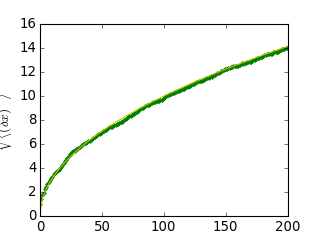We find a well-known result in physics: the RMS distance grows as the square root of the time!

• Basic operations on numpy arrays (addition, etc.) are elementwise

• This works on arrays of the same size.

Nevertheless, It’s also possible to do operations on arrays of different
sizes if Numpy can transform these arrays so that they all have
the same size: this conversion is called broadcasting.

The image below gives an example of broadcasting: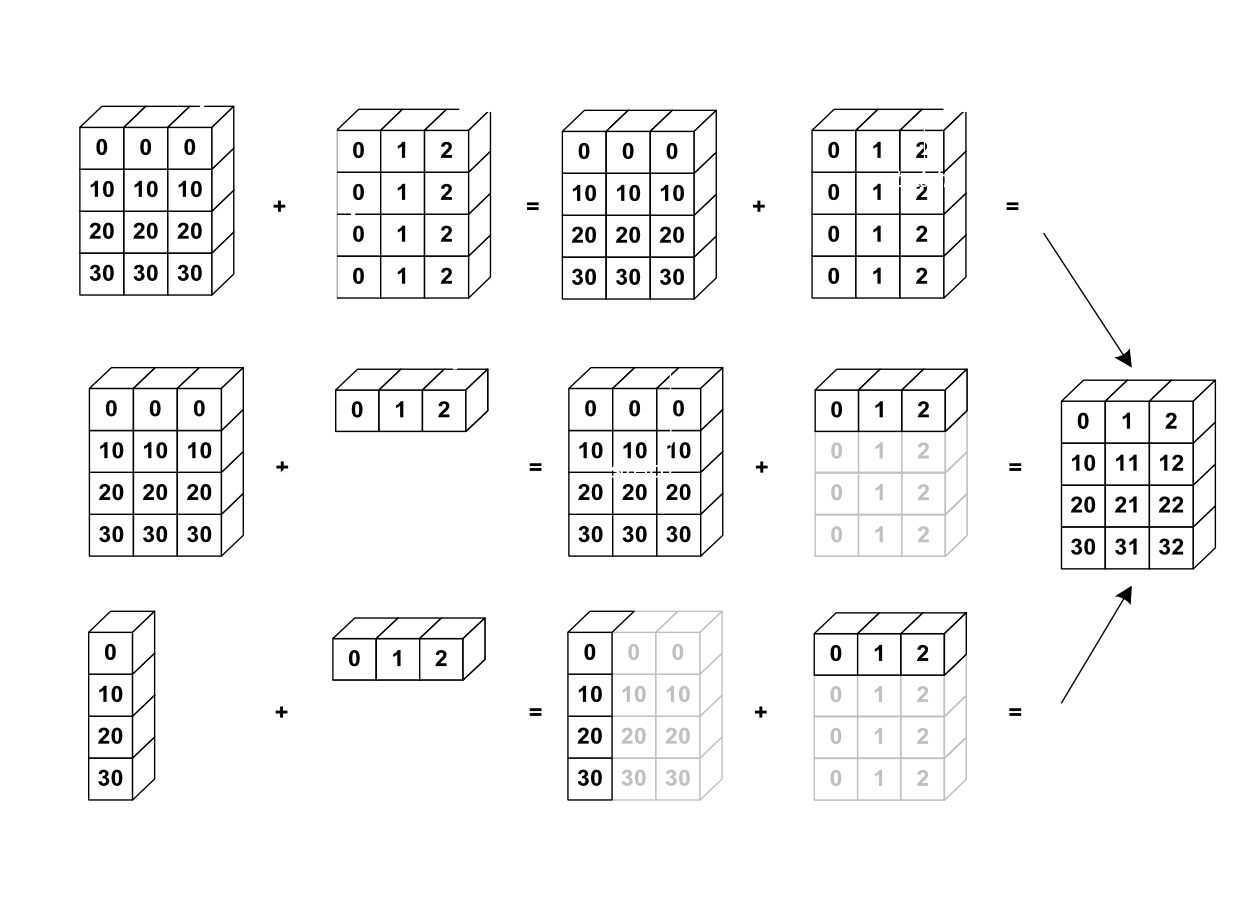Let’s verify:

>>> a = np.tile(np.arange(0, 40, 10), (3, 1)).T
>>> a
array([[ 0,  0,  0],
[10, 10, 10],
[20, 20, 20],
[30, 30, 30]])
>>> b = np.array([0, 1, 2])
>>> a + b
array([[ 0,  1,  2],
[10, 11, 12],
[20, 21, 22],
[30, 31, 32]])


>>> a = np.ones((4, 5))
>>> a = 2  # we assign an array of dimension 0 to an array of dimension 1
>>> a
array([[ 2.,  2.,  2.,  2.,  2.],
[ 1.,  1.,  1.,  1.,  1.],
[ 1.,  1.,  1.,  1.,  1.],
[ 1.,  1.,  1.,  1.,  1.]])


An useful trick:

>>> a = np.arange(0, 40, 10)
>>> a.shape
(4,)
>>> a = a[:, np.newaxis]  # adds a new axis -> 2D array
>>> a.shape
(4, 1)
>>> a
array([[ 0],
,
,
])
>>> a + b
array([[ 0,  1,  2],
[10, 11, 12],
[20, 21, 22],
[30, 31, 32]])


Tip

Broadcasting seems a bit magical, but it is actually quite natural to use it when we want to solve a problem whose output data is an array with more dimensions than input data.

Let’s construct an array of distances (in miles) between cities of Route 66: Chicago, Springfield, Saint-Louis, Tulsa, Oklahoma City, Amarillo, Santa Fe, Albuquerque, Flagstaff and Los Angeles.

>>> mileposts = np.array([0, 198, 303, 736, 871, 1175, 1475, 1544,
...        1913, 2448])
>>> distance_array = np.abs(mileposts - mileposts[:, np.newaxis])
>>> distance_array
array([[   0,  198,  303,  736,  871, 1175, 1475, 1544, 1913, 2448],
[ 198,    0,  105,  538,  673,  977, 1277, 1346, 1715, 2250],
[ 303,  105,    0,  433,  568,  872, 1172, 1241, 1610, 2145],
[ 736,  538,  433,    0,  135,  439,  739,  808, 1177, 1712],
[ 871,  673,  568,  135,    0,  304,  604,  673, 1042, 1577],
[1175,  977,  872,  439,  304,    0,  300,  369,  738, 1273],
[1475, 1277, 1172,  739,  604,  300,    0,   69,  438,  973],
[1544, 1346, 1241,  808,  673,  369,   69,    0,  369,  904],
[1913, 1715, 1610, 1177, 1042,  738,  438,  369,    0,  535],
[2448, 2250, 2145, 1712, 1577, 1273,  973,  904,  535,    0]])A lot of grid-based or network-based problems can also use broadcasting. For instance, if we want to compute the distance from the origin of points on a 10x10 grid, we can do

>>> x, y = np.arange(5), np.arange(5)[:, np.newaxis]
>>> distance = np.sqrt(x ** 2 + y ** 2)
>>> distance
array([[ 0.        ,  1.        ,  2.        ,  3.        ,  4.        ],
[ 1.        ,  1.41421356,  2.23606798,  3.16227766,  4.12310563],
[ 2.        ,  2.23606798,  2.82842712,  3.60555128,  4.47213595],
[ 3.        ,  3.16227766,  3.60555128,  4.24264069,  5.        ],
[ 4.        ,  4.12310563,  4.47213595,  5.        ,  5.65685425]])


Or in color:

>>> plt.pcolor(distance)
>>> plt.colorbar()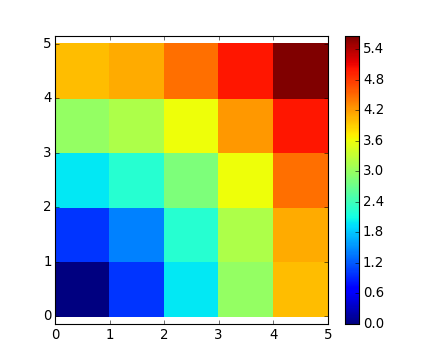Remark : the numpy.ogrid function allows to directly create vectors x and y of the previous example, with two “significant dimensions”:

>>> x, y = np.ogrid[0:5, 0:5]
>>> x, y
(array([,
,
,
,
]), array([[0, 1, 2, 3, 4]]))
>>> x.shape, y.shape
((5, 1), (1, 5))
>>> distance = np.sqrt(x ** 2 + y ** 2)


Tip

So, np.ogrid is very useful as soon as we have to handle computations on a grid. On the other hand, np.mgrid directly provides matrices full of indices for cases where we can’t (or don’t want to) benefit from broadcasting:

>>> x, y = np.mgrid[0:4, 0:4]
>>> x
array([[0, 0, 0, 0],
[1, 1, 1, 1],
[2, 2, 2, 2],
[3, 3, 3, 3]])
>>> y
array([[0, 1, 2, 3],
[0, 1, 2, 3],
[0, 1, 2, 3],
[0, 1, 2, 3]])


## 1.3.2.4. Array shape manipulation¶

### 1.3.2.4.1. Flattening¶

>>> a = np.array([[1, 2, 3], [4, 5, 6]])
>>> a.ravel()
array([1, 2, 3, 4, 5, 6])
>>> a.T
array([[1, 4],
[2, 5],
[3, 6]])
>>> a.T.ravel()
array([1, 4, 2, 5, 3, 6])


Higher dimensions: last dimensions ravel out “first”.

### 1.3.2.4.2. Reshaping¶

The inverse operation to flattening:

>>> a.shape
(2, 3)
>>> b = a.ravel()
>>> b = b.reshape((2, 3))
>>> b
array([[1, 2, 3],
[4, 5, 6]])


Or,

>>> a.reshape((2, -1))    # unspecified (-1) value is inferred
array([[1, 2, 3],
[4, 5, 6]])


Warning

ndarray.reshape may return a view (cf help(np.reshape))), or copy

Tip

>>> b[0, 0] = 99
>>> a
array([[99,  2,  3],
[ 4,  5,  6]])


Beware: reshape may also return a copy!:

>>> a = np.zeros((3, 2))
>>> b = a.T.reshape(3*2)
>>> b = 9
>>> a
array([[ 0.,  0.],
[ 0.,  0.],
[ 0.,  0.]])


Indexing with the np.newaxis object allows us to add an axis to an array (you have seen this already above in the broadcasting section):

>>> z = np.array([1, 2, 3])
>>> z
array([1, 2, 3])

>>> z[:, np.newaxis]
array([,
,
])

>>> z[np.newaxis, :]
array([[1, 2, 3]])


### 1.3.2.4.4. Dimension shuffling¶

>>> a = np.arange(4*3*2).reshape(4, 3, 2)
>>> a.shape
(4, 3, 2)
>>> a[0, 2, 1]
5
>>> b = a.transpose(1, 2, 0)
>>> b.shape
(3, 2, 4)
>>> b[2, 1, 0]
5


Also creates a view:

>>> b[2, 1, 0] = -1
>>> a[0, 2, 1]
-1


### 1.3.2.4.5. Resizing¶

Size of an array can be changed with ndarray.resize:

>>> a = np.arange(4)
>>> a.resize((8,))
>>> a
array([0, 1, 2, 3, 0, 0, 0, 0])


However, it must not be referred to somewhere else:

>>> b = a
>>> a.resize((4,))
Traceback (most recent call last):
File "<stdin>", line 1, in <module>
ValueError: cannot resize an array that has been referenced or is
referencing another array in this way.  Use the resize function
Traceback (most recent call last):
File "<stdin>", line 1, in <module>
ValueError: cannot resize an array that has been referenced or is


Exercise: Shape manipulations

• Look at the docstring for reshape, especially the notes section which has some more information about copies and views.
• Use flatten as an alternative to ravel. What is the difference? (Hint: check which one returns a view and which a copy)
• Experiment with transpose for dimension shuffling.

## 1.3.2.5. Sorting data¶

Sorting along an axis:

>>> a = np.array([[4, 3, 5], [1, 2, 1]])
>>> b = np.sort(a, axis=1)
>>> b
array([[3, 4, 5],
[1, 1, 2]])


Note

Sorts each row separately!

In-place sort:

>>> a.sort(axis=1)
>>> a
array([[3, 4, 5],
[1, 1, 2]])


Sorting with fancy indexing:

>>> a = np.array([4, 3, 1, 2])
>>> j = np.argsort(a)
>>> j
array([2, 3, 1, 0])
>>> a[j]
array([1, 2, 3, 4])


Finding minima and maxima:

>>> a = np.array([4, 3, 1, 2])
>>> j_max = np.argmax(a)
>>> j_min = np.argmin(a)
>>> j_max, j_min
(0, 2)


Exercise: Sorting

• Try both in-place and out-of-place sorting.
• Try creating arrays with different dtypes and sorting them.
• Use all or array_equal to check the results.
• Look at np.random.shuffle for a way to create sortable input quicker.
• Combine ravel, sort and reshape.
• Look at the axis keyword for sort and rewrite the previous exercise.

## 1.3.2.6. Summary¶

What do you need to know to get started?

• Know how to create arrays : array, arange, ones, zeros.

• Know the shape of the array with array.shape, then use slicing to obtain different views of the array: array[::2], etc. Adjust the shape of the array using reshape or flatten it with ravel.

• Obtain a subset of the elements of an array and/or modify their values with masks

>>> a[a < 0] = 0

• Know miscellaneous operations on arrays, such as finding the mean or max (array.max(), array.mean()). No need to retain everything, but have the reflex to search in the documentation (online docs, help(), lookfor())!!

• For advanced use: master the indexing with arrays of integers, as well as broadcasting. Know more Numpy functions to handle various array operations.